# Glossary

A glossary of general terms

## Coefficient of Determination, Determination Coefficient, RSquared,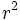$r^2$

The coefficient of determination, or$r^2$ value, is a statistical measure computed over a pair of sample distributions, giving a measure of how strongly the means of the two distributions differ in relation to variance. In a BCI context, the coefficient of determination is computed over signals that have been measured under two different task conditions, and represents the fraction of the total signal variance that is accounted for ("determined by") by the task condition. It is a measure of how well the original task condition ("user intent") may be inferred from a brain signal.

### Computation

The coefficient of determination is in fact the squared correlation coefficient for a single bivariate distribution constructed from two sets of univariate data as follows:

• There are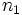$n_1$ values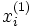$x^{(1)}_i$ measured under condition 1, and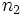$n_2$ values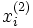$x^{(2)}_i$ measured under condition 2.
• From these two data sets, a single, two-dimensional data set is constructed, consisting of points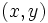$(x,y)$ by assigning the measured value to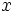$x$, and setting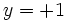$y=+1$ if the value has been measured under condition 1, and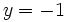$y=-1$ for condition 2. The actual label values assigned to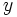$y$ do not matter, as long as they are distinct, but choosing +1 and -1 simplifies further computation.
• For any two-dimensional data set of points$(x,y)$, the squared correlation coefficient is: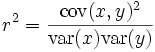$r^2=\frac{\textrm{cov}(x,y)^2}{\textrm{var}(x) \textrm{var}(y)}$.

In the special case of the distribution defined above, defining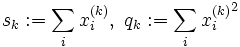$s_k:=\sum_i x^{(k)}_i,\ q_k:=\sum_i {x^{(k)}_i}^2$,

we have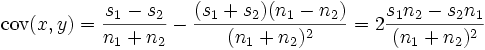$\textrm{cov}(x,y) = \frac{s_1-s_2}{n_1+n_2}-\frac{(s_1+s_2)(n_1-n_2)}{(n_1+n_2)^2}=2\frac{s_1n_2-s_2n_1}{(n_1+n_2)^2}$,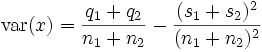$\textrm{var}(x) = \frac{q_1 +q_2}{n_1+n_2}-\frac{(s_1 + s_2)^2}{(n_1+n_2)^2}$,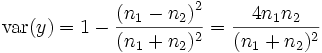$\textrm{var}(y) = 1-\frac{\left(n_1-n_2\right)^2}{(n_1+n_2)^2} = \frac{4n_1n_2}{(n_1+n_2)^2}$,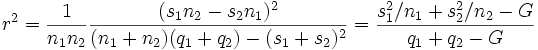$r^2 = \frac{1}{n_1n_2} \frac{(s_1n_2-s_2n_1)^2}{(n_1+n_2)(q_1+q_2)-(s_1+s_2)^2} = \frac{{s_1^2}/{n_1}+{s_2^2}/{n_2}-G}{q_1+q_2-G}$

where we have introduced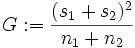$G:=\frac{(s_1+s_2)^2}{n_1+n_2}$ to arrive at the last expression, which is useful for efficient computation of$r^2$.

### Relation to Classifier Training, and Linear Discriminant Analysis (LDA)

LDA is a training method for linear classifiers. A linear classifier is a classification algorithm that infers the condition (class, task, label) under which a point of data was recorded, simply by computing a weighted sum of the data point's dimensions, and comparing the result to a threshold. "Training" a classifier means that recorded data is used in order to determine a weighting that is "optimally suited" to do so. In high-dimensional space, weighting dimensions corresponds to the geometrical operation of projecting a data point onto a line. Thus, one also speaks of a "linear projection".

In case of LDA, the criterion of optimality is chosen as the ratio of "inter-class variance" and "within-class variance". The LDA algorithm determines a projection from high-dimensional data space onto a single dimension such that, for the resulting numbers, the ratio of mean difference between classes, and variance within classes, is as large as possible. Comparing this ratio to the above formulae, you may see that the numerator of the$r^2$ value is proportional to the squared mean difference between classes, and the denominator is proportional to the squared mean difference within each class (when assuming equal covariances, as LDA does in its simplest form).

Thus, we may say that LDA classifier training chooses a linear classifier such that the classifier's output has the highest possible$r^2$ value, given the data available.

### Significance Test

For small numbers of trials (<30 per label), determination coefficients can become rather large even if there is no significant effect. To assess significance under the (robust) assumption of Gaussianity, determination coefficients may be converted into t-values according to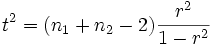$t^2=(n_1 + n_2 -2) \frac{r^2}{1-r^2}$.

Such a t-value may be used in a one-tailed t-test against the "no correlation" null hypothesis.

## Sensorimotor Rhythm (SMR)

A general term referring to idling activity from the sensory and motor cortices. The mu rhythm is the most prominent SMR activity.